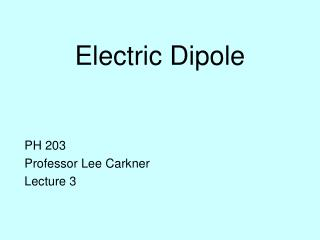DownloadDownload PresentationElectric Dipole

# Electric Dipole

Télécharger la présentation## Electric Dipole

- - - - - - - - - - - - - - - - - - - - - - - - - - - E N D - - - - - - - - - - - - - - - - - - - - - - - - - - -
##### Presentation Transcript

1. Electric Dipole PH 203 Professor Lee Carkner Lecture 3

2. EB PAL # 2 Electric Field q q EA • Distance to point P is 5 cm (hypotenuse of a 3-4-5 right triangle) • Top angle of triangle, sin q = 3/5, q = 37 deg. • EA = kq/r2 = (8.99X109)(5X10-6) / (0.05)2 = 1.8X107 N/C • qA = 270 - q = 233 deg • EB = (8.99X109)(2)(5X10-6) / (0.05)2 = 3.6X107 N/C • qB = q + 90 = 127 deg +2q

3. PAL # 2 Electric Field E +2q • EAX = EA cos qA = (1.8X107)(cos 233) = -1.1X107 N/C • EAY = EA sin qA = (1.8X107)(sin 233) = -1.4X107 N/C • EBX =EB cos qB = (3.6X107)(cos 127) = -2.2X107 N/C • EBY = EB sin qB = (3.6X107)(sin 127) = 2.9X107 N/C • EX = EAX + EBX = -3.3X107 N/C • EY = EAY + EBY = 1.5X107 N/C • E2 = EX2 + EY2 • E = 3.6X107 N/C • q = arctan (EY/EX) = 24 deg above negative x-axis • q = 180-22 =156 deg from positive x-axis

4. The Dipole • Dipole moment = p = qd • z is the distance from the center of the dipole to some point on the dipole axis

5. Dipole Field • At a distance z that is large compared to d, the electric field reduces to: E = (1/(2pe0)) (p/z3) • Note that: • E falls off very rapidly with z • Doubling charge is the same as doubling distance between charges

6. Dipole in an External Field • Assumptions: • The dipole’s structure is rigid and unchangeable • Since the two charges are equal and opposite, the two forces are equal and opposite • However, since the charges are not in the same place, there is a net torque on the dipole • A dipole in an external field will have no translation motion, but will have rotational motion

7. Dipole Torque • Torque is then Fd sin q = Eqd sin q • Remember that p is direction from negative charge to positive charge

8. How will the dipole spin? • The dipole wants to align the dipole moment with E • Dipole has the most torque when perpendicular to the field (q = 90)

9. Dipole Energy • We set the potential energy (U) to be zero when the dipole is at right angles to the field (q= 90) • Dipole perpendicular to field: U = 0 • Can write potential energy as: • The work (done by an external force) to turn a dipole in a field is thus: W = Uf - Ui

10. Charge Distribution • The charge density: • Linear = l = C/m • Surface = • Volume = r =C/m3 • For example: dE = (1/(4pe0)) (lds/r2)

11. Rings • For a uniform charged ring: E = qz / (4pe0(z2+R2)3/2)

12. Disks • For a uniform charged disk: E = (s/2e0)(1 –[z/(z2+R2)½] • The field depends not on the total charge but the charge density

13. Next Time • Read 23.1-23.4 • Problems: Ch 22, P: 42, 52, 53, Ch 23, P: 4, 5

14. What is true about the magnitude and direction of the fields from charges A and B at point P? • They are equal in magnitude and point in the same direction • They are equal in magnitude and point towards charges A and B • They are unequal in magnitude and point away from charges A and B • They are unequal in magnitude and 180 apart in direction • The net field at P is zero A B

15. What is true about the x and y components of the fields from charges A and B at point P? • They both add • They both cancel • The x components add and the y components cancel • The x components cancel and the y components add • We can’t tell with out knowing the magnitude of q A B

16. The above electric field, • increases to the right • increases to the left • increases up • increases down • is uniform

17. An electron placed at A, • Would move left and feel twice the force as an electron at B • Would move right and feel twice the force as an electron at B • Would move left and feel half the force as an electron at B • Would move right and feel half the force as an electron at B • Would move right and feel the same force as an electron at B